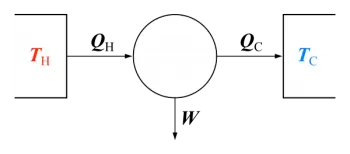# What Is Ionization Enthalpy? Enthalpy Definition and TypesEnthalpy is the amount of energy that a thermodynamic system can exchange with its surroundings. Enthalpy is also known as absolute enthalpy or heat of reaction and is represented by the letter H.

As defined, enthalpy itself encompasses not only the internal energy of the system but also the energy stored in the system's environment (surroundings).

In short, enthalpy is the sum of the internal energy of matter and the product of its volume times the pressure according to the following formula: H = Einternal + P·V. Thus, it depends on the pressure and the volume of the system.

This property is a quantifiable state function. Hence, although the total system's enthalpy cannot be measured directly, we can measure the ability to receive or contribute energy to a system.

The enthalpy of a system is expressed in Joules international system of measures. Although the amount of heat exchanged can also be expressed in kilocalories (kcal).

The first scientist who defined this term was the Dutch physicist Heike Kamerlingh Onnes, Nobel Prize in Physics in 1913.

## What Is the Ionization Enthalpy?

Ionisation enthalpy, also called ionization potential, is the energy required to remove an electron from the outer shells of a neutral, gaseous atom in its ground state.

This energy is which corresponds to the first ionization energy.

The second ionization enthalpy is the energy needed to remove a second outermost electron. Its value is always more significant than the first since the volume of a positive ion is less than that of the neutral atom. The attractive force is more potent in the positive ion than in the neutral atom.

As you can imagine, the following ionization enthalpies keep increasing until the atom is empty of electrons.

## What Is the Enthalpy of Reaction?

The standard enthalpy of reaction is the enthalpy change associated with a chemical reaction between elements and compounds under standard conditions in its most stable form at the temperature of interest and the standard state pressure, defined in many cases as 1 bar.

Its value is negative if it is associated with an exothermic reaction. In this case, it indicates the energy released. On the other hand, the value is positive if associated with an endothermic reaction.

## What Is the Enthalpy of Formation?

The standard enthalpy of formation of a compound at a given temperature is the change in heat absorbed or released during the formation of a quantity of that substance in its standard state.

All elements in their normal state, thus pure in the most stable form at the temperature of interest and the standard state pressure, have zero as their standard value.

If the reaction to form the compound is exothermic, the value is negative. On the contrary, if the reaction is endothermic, the value is positive.

## What Is Bond Enthalpy?

We define bond enthalpy as the amount of energy required to create or break a chemical bond between two chemical elements at constant pressure.

More formally, we can define the standard bond enthalpy as the variation of heat released or absorbed, under standard conditions of one atmosphere and 25 degrees Celsius, that accompanies the formation reaction of one mole of chemical bonds from isolated atoms in a gaseous state.

## Other Types of Enthalpy

• Enthalpy of formation is the energy required to form one mole of a substance from its component elements.

• Enthalpy of reaction is the energy released by a chemical reaction subjected to constant pressure.

• Enthalpy of solution refers to the amount of heat given up or absorbed by a substance when dissolved in an aqueous solution.

• Enthalpy of neutralization is the energy captured or released when an acid and a base are mixed, neutralizing each other.

• Enthalpy of combustion is the energy released when one mole of organic substance reacts with oxygen in the air and releases carbon dioxide (CO2).

• Enthalpy of decomposition is the amount of heat or energy released when a mole of a substance is broken down into simpler elements.

• Enthalpy of dissolution refers to the amount of heat or energy that a substance absorbs or gives up when more water is added to the solution.

• Enthalpy of fusion is the enthalpy change in the transition from a solid to a liquid state.

• Sublimation enthalpy: the change in enthalpy in the transition from solid to gas.

• Enthalpy of evaporation is the energy needed to passage a substance from liquid to gas.

## What Is the Difference Between Enthalpy and Entropy?

Entropy quantifies the degree of disorder of a thermodynamic system. On the other hand, enthalpy represents the total energy contained within a thermodynamic system. Therefore, it is the energy that the system possesses that it can release.

However, both values ​​are related through temperature: The enthalpy change equals the entropy change multiplied by the temperature.

Author:

Published: December 19, 2017
Last review: March 26, 2022